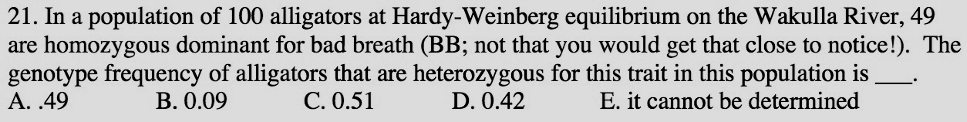# Problem: In a population of 100 alligators at Hardy-Weinberg equilibrium on the Wakulla River, 49 are homozygous dominant for bad breath(BB; not that you would get that close to notice). The genotype frequency of alligators that are heterozygous for this trait in this population is _____.A. 49B 0.09C. 0.51D. 0.42E. it cannot be determined

###### FREE Expert Solution

If a population is at Hardy Weinberg equilibrium, it follows the equation for the allele frequency, p + q = 1, where p is the relative frequency of the dominant allele that is present in the population, while q is the relative frequency for the recessive allele. In addition to this, the trait in question would have a constant frequency for the genotypes for the trait.###### Problem Details

In a population of 100 alligators at Hardy-Weinberg equilibrium on the Wakulla River, 49 are homozygous dominant for bad breath(BB; not that you would get that close to notice). The genotype frequency of alligators that are heterozygous for this trait in this population is _____.

A. 49

B 0.09

C. 0.51

D. 0.42

E. it cannot be determined